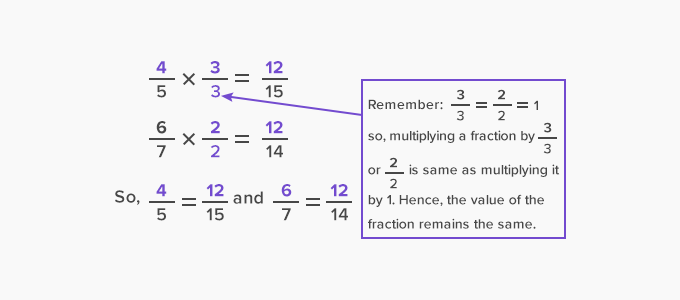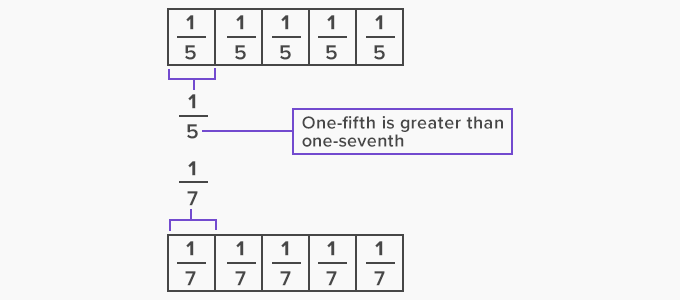# Common Numerator - Definition with Examples

The Complete K-5 Math Learning Program Built for Your Child

• 30 Million Kids

Loved by kids and parent worldwide

• 50,000 Schools

Trusted by teachers across schools

• Comprehensive Curriculum

Aligned to Common Core

What is Common Numerator?

A non-zero number that is a multiple of the numerators of two or more fractions is called their common numerator.

For example, consider the fractions 4and 6. Both the fractions have different numerators. To find their common numerator, we find the common multiples of the numerators 4 and 6.

Multiples of 4: 4, 8, 12, 16, 20, 24, 28, 32, 36, 40, ...

Multiples of 6: 6, 12, 18, 24, 30, 36, 42, ...

Common multiples of 4 and 6: 12, 24, 36, …

So, common numerators for 4and 6can be 12, 24, 36, …

If we want the common numerator to be 12, we need to multiply both the numerator and denominator as shown:Now, we have two fractions with a common numerator.When two or more fractions have a common numerator, we say their numerators are like.

Comparing fractions with common numerator

We can easily compare two or more fractions when their numerators are common or like.

For example, let us compare 3and 3⁄5

As fifths are greater than sevenths, three-fifths are greater than three-sevenths.Therefore, 3< 35

So, in general:

If two or more fractions have a common numerator then the fraction with the smallest denominator is the greatest.

 Fun Facts The word numerator is derived from the Latin word “numerātor” which means “counter”.

Won Numerous Awards & Honors